# Mechanical Engineering - Hydraulics and Fluid Mechanics

### Exercise :: Hydraulics and Fluid Mechanics - Section 6

21.

The depth of centre of pressure (h) for a vertically immersed surface from the liquid surface is given by (where IG = Moment of inertia of the immersed surface about horizontal axis through its centre of gravity, A = Area of immersed surface, and x = Depth of centre of gravity of the immersed surface from the liquid surface)

 A.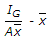B.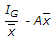C.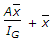D.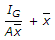Answer: Option D

Explanation:

No answer description available for this question. Let us discuss.

22.

The velocity at which the turbulent flow starts is known as higher critical velocity.

 A. Yes B. No

Answer: Option A

Explanation:

No answer description available for this question. Let us discuss.

23.

According to Francis formula, the discharge over a rectangular weir is (where n = Number of end contractions)

 A.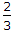Cd(L - nH)2gH B.Cd(L - 0.1nH)2gH3/2 C.Cd(L - nH)2gH2 D.Cd(L - 0.2nH)2gH5/2

Answer: Option B

Explanation:

No answer description available for this question. Let us discuss.

24.

The specific weight is also known as weight density.

 A. Correct B. Incorrect

Answer: Option A

Explanation:

No answer description available for this question. Let us discuss.

25.

In a lockgate, the reaction between two gates is (where P = Resultant pressure on the lock gate, and α = Inclination of the gate with the normal to the side of the lock)

 A.B.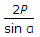C. P / 2sinα D.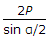Answer: Option C

Explanation:

No answer description available for this question. Let us discuss.

#### Current Affairs 2021

Interview Questions and Answers